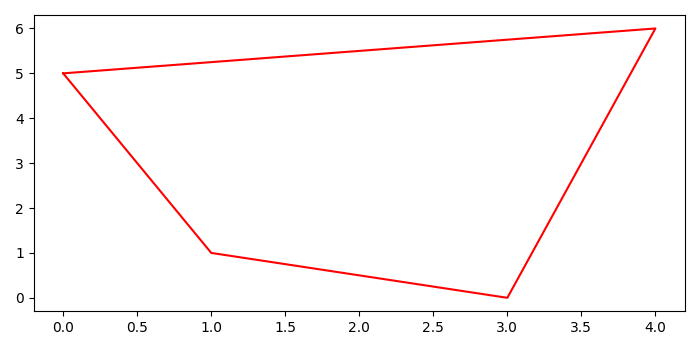# How do I plot Shapely polygons and objects using Matplotlib?

To plot shapely polygons and objects using matplotlib, the steps are as follows −

• Create a polygon object using (x, y) data points.

• Get x and y, the exterior data, and the array using polygon.exterior.xy.

• Plot x and y data points using plot() method with red color.

## Example

from shapely.geometry import Polygon
import matplotlib.pyplot as plt
plt.rcParams["figure.figsize"] = [7.00, 3.50]
plt.rcParams["figure.autolayout"] = True
polygon1 = Polygon([(0, 5),
(1, 1),
(3, 0),
(4, 6),
])
x, y = polygon1.exterior.xy
plt.plot(x, y, c="red")
plt.show()

## Output